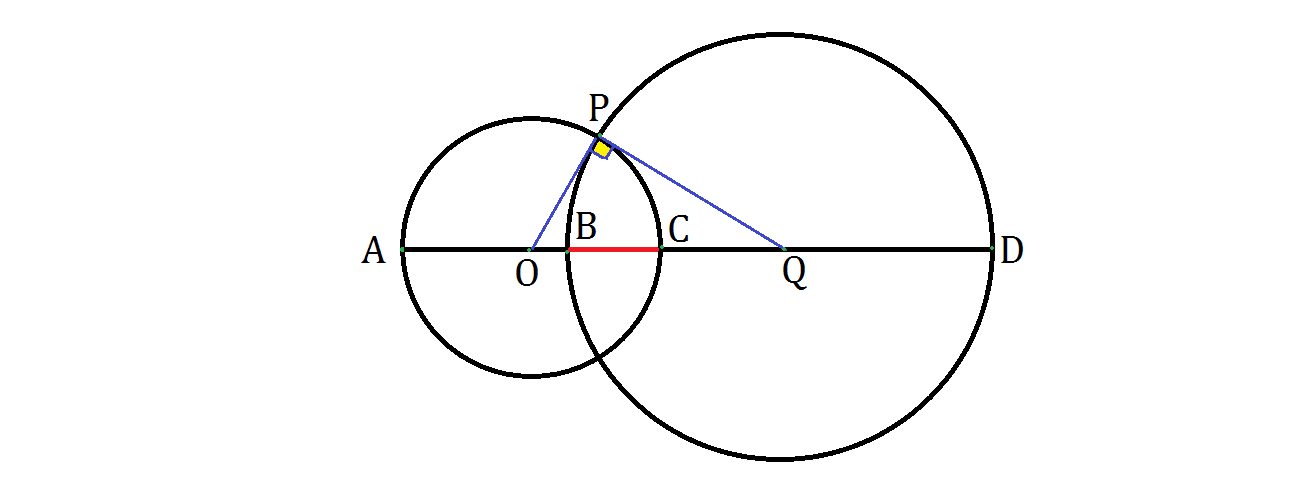# Orthogonal CirclesTwo circles $O$ and $Q$ are orthogonal: their radii are perpendicular at their intersection point $P,$ as shown above. Then the line $AD$ is drawn such that it passes through both centers and intersects the arcs at points $B$ and $C.$

If $AB = 10$ and $CD = 24$, what is the length of the red segment $BC?$

×